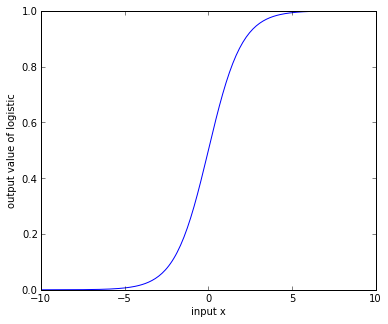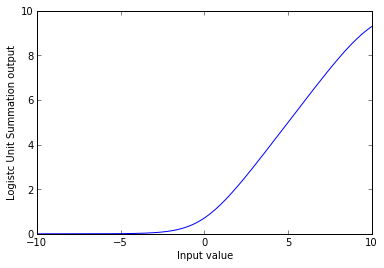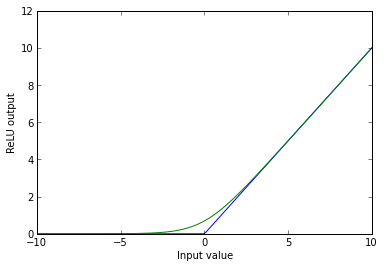-->

## Friday, January 10, 2014

### Rectified Linear Units are just the Summation of Many Sigmoids

I was watching Geoff Hinton's lecture from May 2013 about the history of deep learning and his comments on the rectified linear units (ReLU's) made more sense that my previous reading on them had. Essentially he noted that these units were just a way of approximating the activity of a large number of sigmoid units with varying biases.

"Is that true?" I wondered. "Let's try it and see ... "

In :
%pylab inline


Welcome to pylab, a matplotlib-based Python environment [backend: module://IPython.zmq.pylab.backend_inline].



Let's first define a logistic sigmoid unit. This looks like

$f(x;\alpha) = \frac{1}{1+ \exp(-x + \alpha)}$

where $\alpha$ is the offset paramter to set the value at which the logistic evaluates to 0. Programatically this looks like:

In :
def logistic(x,offset):
# X is an array of numbers at which to evaluate the logistc unit, offset is the offset value
return (1/(1 + np.exp(-x+offset)))


When evaluated for a number of values we see a distinctive 's'-shape. As the limits of the function evaluation expand this shape becomes much more 'squashed.' This is one of the difficulties of using such a function to limit the input values to a learning system. If you're unsure of the range of values to be inputted then the output can easily saturate for very large or very small values. Input normalization can help this, but sometimes this isn't practical (e.g. if you have a bimodal input distribution with heavy tails.) The $\alpha$ parameter lets you adjust for this.

In :
x = np.linspace(-10,10,200)
y = logistic(x,0)
fig = plot(x,y)
xlabel('input x')
ylabel('output value of logistic')
gcf().set_size_inches(6,5)So what happens if you were to sum many of these functions, all with a different bias?

In :
N_sum = 10      # Number of logistics to sum
offsets = np.linspace(0,np.max(x),100)
y_sum = np.zeros(shape(x))
for offset in offsets:
y_sum += logistic(x,offset)

y_sum = y_sum / N_sum

In :
plot(x,y_sum)
xlabel('Input value')
ylabel('Logistc Unit Summation output')

Out:
<matplotlib.text.Text at 0x10699bed0>Yep, that definitely is starting to look like the ReLU's.

So it turns out that you can approximate this summation using the equation

$f(x) = \log(1+exp(x))$

In :
x = np.linspace(-10,10,200)
inp = np.linspace(-10,10,200)
inp[inp < 0] = 0
f2 = plot(x,inp)
xlabel('Input value')
ylabel('ReLU output')

relu_approx = np.log(1 + np.exp(x))
f3 = plot(x,relu_approx)From here Hinton said, "do you really need the 'log' and the 'exp', or could I just take $max(0,input)$? And that works fine", thus giving you the ReLU.

Hinton's discussion is embedded below. He starts talking about different learning units at 27 minutes, 10 seconds.

In :
from IPython.display import YouTubeVideo